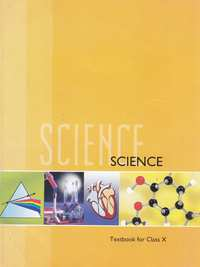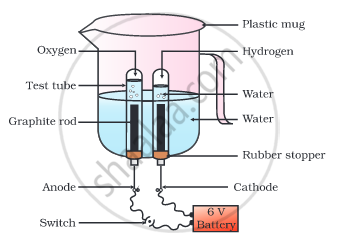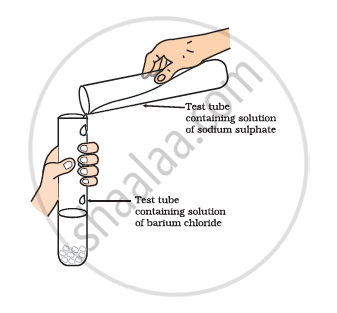# NCERT solutions for Class 10 Science chapter 1 - Chemical Reactions and Equations [Latest edition]

#### Chapters## Solutions for Chapter 1: Chemical Reactions and Equations

Below listed, you can find solutions for Chapter 1 of CBSE NCERT for Class 10 Science.

Intext QuestionsIntext QuestionsIntext QuestionsExercises
Intext Questions [Page 6]

### NCERT solutions for Class 10 Science Chapter 1 Chemical Reactions and Equations Intext Questions [Page 6]

Intext Questions | Q 1 | Page 6

Why should a magnesium ribbon be cleaned before it is burnt in air?

Intext Questions | Q 2.1 | Page 6

Write the balanced equation for the following chemical reaction

Hydrogen + Chlorine → Hydrogen chloride

Intext Questions | Q 2.2 | Page 6

Write the balanced equation for the following chemical reaction

Barium chloride + Aluminium sulphate → Barium sulphate + Aluminium chloride

Intext Questions | Q 2.3 | Page 6

Write the balanced equation for the following chemical reaction

Sodium + Water → Sodium hydroxide + Hydrogen

Intext Questions | Q 3.1 | Page 6

Write a balanced chemical equation with state symbols for the following reaction.

Solutions of barium chloride and sodium sulphate in water react to give insoluble barium sulphate and the solution of sodium chloride.

Intext Questions | Q 3.2 | Page 6

Write a balanced chemical equation with state symbols for the following reaction.

Sodium hydroxide solution (in water) reacts with hydrochloric acid solution (in water) to produce sodium chloride solution and water.

Intext Questions [Page 10]

### NCERT solutions for Class 10 Science Chapter 1 Chemical Reactions and Equations Intext Questions [Page 10]

Intext Questions | Q 1 | Page 10

A solution of a substance ‘X’ is used for white washing.
(i) Name the substance ‘X’ and write its formula.
(ii) Write the reaction of the substance ‘X’ named in (i) above with water.

Intext Questions | Q 2 | Page 10

Why is the amount of gas collected in one of the test tubes in the following Activity double of the amount collected in the other? Name this gas.

• Take a plastic mug. Drill two holes at its base and fit rubber stoppers in these holes. Insert carbon electrodes in these rubber stoppers as shown in the following Fig.
• Connect these electrodes to a 6 volt battery.
• Fill the mug with water such that the electrodes are immersed. Add a few drops of dilute sulphuric acid to the water.
• Take two test tubes filled with water and invert them over the two carbon electrodes.
• Switch on the current and leave the apparatus undisturbed for some time.
• You will observe the formation of bubbles at both the electrodes. These bubbles displace water in the test tubes.
• Is the volume of the gas collected the same in both the test tubes?
• Once the test tubes are filled with the respective gases, remove them carefully.
• Test these gases one by one by bringing a burning candle close to the mouth of the test tubes.Intext Questions [Page 13]

### NCERT solutions for Class 10 Science Chapter 1 Chemical Reactions and Equations Intext Questions [Page 13]

Intext Questions | Q 1 | Page 13

Why does the colour of copper sulphate solution change when an iron nail is dipped in it?

Intext Questions | Q 2 | Page 13

Give an example of a double displacement reaction other than the one given in the following Activity.
•Take about 3 mL of sodium sulphate
solution in a test tube.
• In another test tube, take about 3 mL of
barium chloride solution.
• Mix the two solutions given in the following fig.
• What do you observe?Intext Questions | Q 3 | Page 13

Identify the substances that are oxidised and the substances that are reduced in the following reaction.

4Na (s) + O2 (g) → 2Na2O (s)

Intext Questions | Q 3.2 | Page 13

Identify the substances that are oxidised and the substances that are reduced in the following reaction.

CuO (s) + H2 (g) → Cu (s) + H2O (l)

Exercises [Pages 14 - 16]

### NCERT solutions for Class 10 Science Chapter 1 Chemical Reactions and Equations Exercises [Pages 14 - 16]

Exercises | Q 1 | Page 14

1. Which of the statements about the reaction below are incorrect?
2PbO (s) + C (s) → 2Pb (s) + CO2 (g)
(b) Carbon dioxide is getting oxidised.
(c) Carbon is getting oxidised.
(d) Lead oxide is getting reduced.

• (a) and (b)

• (a) and (c)

• (a), (b) and (c)

• all

Exercises | Q 2 | Page 14

Fe2O3 + 2Al → Al2O3 + 2Fe
The above reaction is an example of a

• combination reaction.

• double displacement reaction.

• decomposition reaction.

• displacement reaction.

Exercises | Q 3 | Page 15

What happens when dilute hydrochloric acid is added to iron fillings? Tick the correct answer.

• Hydrogen gas and Iron chloride are produced.

• Chlorine gas and Iron hydroxide are produced.

• No reaction takes place.

• Iron salt and water are produced.

Exercises | Q 4 | Page 15

What is a balanced chemical equation? Why should a chemical equation be balanced?

Exercises | Q 5.1 | Page 15

Translate the following statements into chemical equations and then balance them.

•  Hydrogen gas combines with nitrogen to form ammonia
Exercises | Q 5.2 | Page 15

Translate the following statements into chemical equations and then balance them.

•  Hydrogen sulphide gas burns in air to give water and sulphur dioxide.
Exercises | Q 5.3 | Page 15

Translate the following statement into chemical equation and then balance them.

• Barium chloride reacts with aluminium sulphate to give aluminium chloride and barium sulphate.
Exercises | Q 5.4 | Page 15

Translate the following statements into chemical equations and then balance them.

• Potassium metal reacts with water to give potassium hydroxide and hydrogen gas
Exercises | Q 6.1 | Page 15

Balance the chemical equation.

HNO3 + Ca(OH)2 Ca(NO3)2 + H2O

Exercises | Q 6.2 | Page 15

Balance the following chemical equation.

NaOH+H2SO4Na2SO4+H2O

Exercises | Q 6.3 | Page 15

Balance the following chemical equation.

NaCl+AgNO3AgCl+NaNO3

Exercises | Q 6.4 | Page 15

Balance the following chemical equation.

BaCl2 + H2SO4 → BaSO4 + HCl

Exercises | Q 7.1 | Page 15

Write the balanced chemical equation for the following reaction.

Calcium hydroxide + Carbon dioxide → Calcium carbonate + Water

Exercises | Q 7.2 | Page 15

Write the balanced chemical equation for the following reaction.

Zinc + Silver nitrate → Zinc nitrate + Silver

Exercises | Q 7.3 | Page 15

Write the balanced chemical equations for the following reaction.

Aluminium + Copper chloride → Aluminium chloride + Copper

Exercises | Q 7.4 | Page 15

Write the balanced chemical equation for the following reaction.

Barium chloride + Potassium sulphate → Barium sulphate + Potassium chloride

Exercises | Q 8.1 | Page 15

Write the balanced chemical equation for the following and identify the type of reaction

Potassium bromide (aq) + Barium iodide (aq) → Potassium iodide (aq) + Barium bromide(s)

Exercises | Q 8.2 | Page 15

Write the balanced chemical equation for the following and identify the type of reaction

Zinc carbonate (s) → Zinc oxide (s) + Carbon dioxide (g)

Exercises | Q 8.3 | Page 15

Write the balanced chemical equation for the following and identify the type of reaction

Hydrogen (g) + Chlorine (g) → Hydrogen chloride (g)

Exercises | Q 8.4 | Page 15

Write the balanced chemical equation for the following and identify the type of reaction

Magnesium (s) + Hydrochloric acid (aq) → Magnesium chloride (aq) + Hydrogen (g)

Exercises | Q 9 | Page 15

What does one mean by endothermic reaction? Give example.

Exercises | Q 10 | Page 15

Why is respiration considered an exothermic reaction? Explain.

Exercises | Q 11 | Page 15

Why are decomposition reactions called the opposite of combination reactions? Write equations for these reactions.

Exercises | Q 12 | Page 16

Write one equation each for decomposition reactions where energy is supplied in the form of heat, light or electricity.

Exercises | Q 13 | Page 16

What is the difference between displacement and double displacement reactions? Write equations for these reactions.

Exercises | Q 14 | Page 16

In the refining of silver, the recovery of silver from silver nitrate solution involved displacement by copper metal. Write down the reaction involved.

Exercises | Q 15 | Page 16

What do you mean by a precipitation reaction? Explain by giving examples.

Exercises | Q 16.1 | Page 16

Explain the following in term of gain or loss of oxygen with two examples.
Oxidation

Exercises | Q 16.2 | Page 16

Explain the following in term of gain or loss of oxygen with two examples.
Reduction

Exercises | Q 17 | Page 16

A shiny brown-coloured element ‘X’ on heating in air becomes black in colour. Name the element ‘X’ and the black coloured compound formed

Exercises | Q 18 | Page 16

Why do we apply paint on iron articles?

Exercises | Q 19 | Page 16

Oil and Fat containing food items are flushed with Nitrogen. Why?

Exercises | Q 20.1 | Page 16

Explain the terms Corrosion

Exercises | Q 20.2 | Page 16

Explain the term Rancidity.

## Solutions for Chapter 1: Chemical Reactions and Equations

Intext QuestionsIntext QuestionsIntext QuestionsExercises## NCERT solutions for Class 10 Science chapter 1 - Chemical Reactions and Equations

Shaalaa.com has the CBSE Mathematics Class 10 Science CBSE solutions in a manner that help students grasp basic concepts better and faster. The detailed, step-by-step solutions will help you understand the concepts better and clarify any confusion. NCERT solutions for Mathematics Class 10 Science CBSE 1 (Chemical Reactions and Equations) include all questions with answers and detailed explanations. This will clear students' doubts about questions and improve their application skills while preparing for board exams.

Further, we at Shaalaa.com provide such solutions so students can prepare for written exams. NCERT textbook solutions can be a core help for self-study and provide excellent self-help guidance for students.

Concepts covered in Class 10 Science chapter 1 Chemical Reactions and Equations are Chemical Equation, Direct Combination (or Synthesis) Reaction, Decomposition Reactions, Types of Chemical Change or Chemical Reaction, Balancing Chemical Equation, Single Displacement Reactions, Double Displacement Reaction, Oxidation, Reduction and Redox Reactions, Corrosion of Metals and Its Prevention, Rancidity of Food and Its Prevention, Chemical Equation, Direct Combination (or Synthesis) Reaction, Decomposition Reactions, Types of Chemical Change or Chemical Reaction, Balancing Chemical Equation, Single Displacement Reactions, Double Displacement Reaction, Oxidation, Reduction and Redox Reactions, Corrosion of Metals and Its Prevention, Rancidity of Food and Its Prevention.

Using NCERT Class 10 Science solutions Chemical Reactions and Equations exercise by students is an easy way to prepare for the exams, as they involve solutions arranged chapter-wise and also page-wise. The questions involved in NCERT Solutions are essential questions that can be asked in the final exam. Maximum CBSE Class 10 Science students prefer NCERT Textbook Solutions to score more in exams.

Get the free view of Chapter 1, Chemical Reactions and Equations Class 10 Science additional questions for Mathematics Class 10 Science CBSE, and you can use Shaalaa.com to keep it handy for your exam preparation.